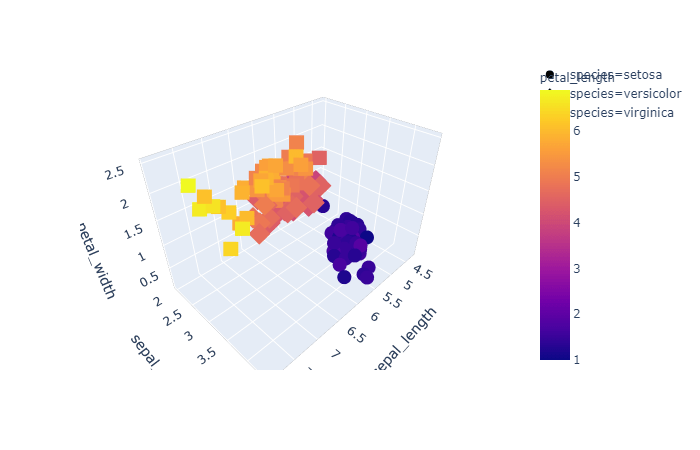# Scatter Plot using Matplotlib in Python

A scatter plot is a type of plot or graph that uses two coordinates axis (say x and y) to display values for two variables for a set of data. We can use matplotlib library of python to create scatter plot in Jupyter notebook. Let us import the required libraries first: #Import the libraries import … Continue reading Scatter Plot using Matplotlib in Python# How to Create 3d Interactive Graph using Plotly

Like matplotlib and seaborn we have plotly, that is a data visualization library used for creating graphical, 3d and interactive graph. You can install plotly using below command pip install plotly Then you can import it in Jupyter notebook as below. import plotly.express as pex Let us use the famous iris dataset to create the … Continue reading How to Create 3d Interactive Graph using Plotly